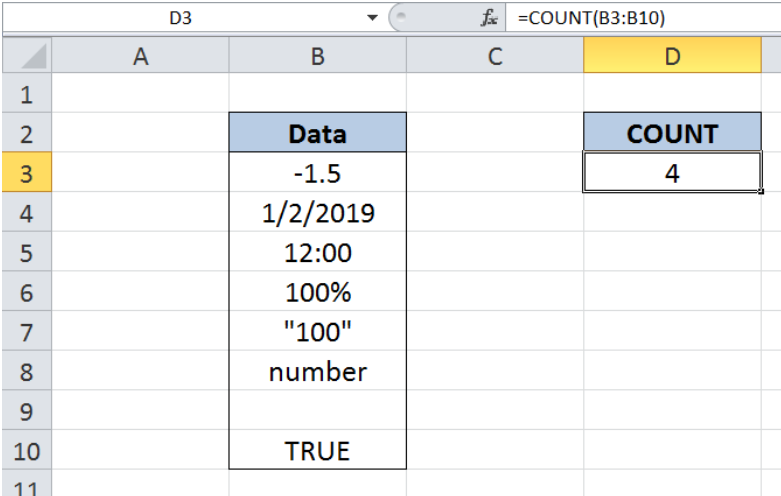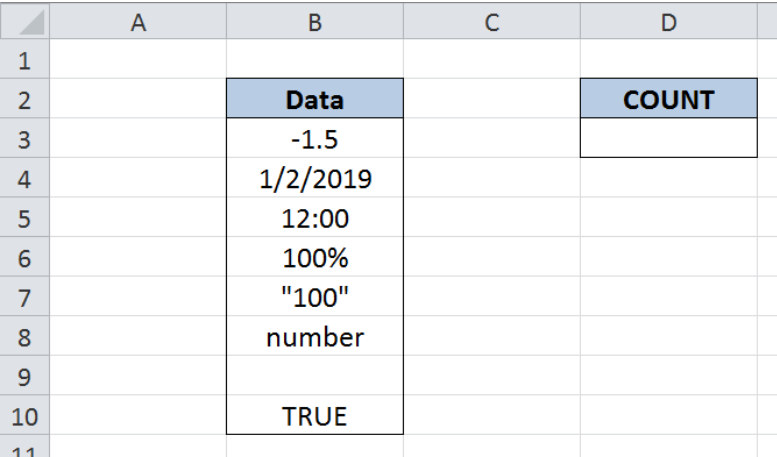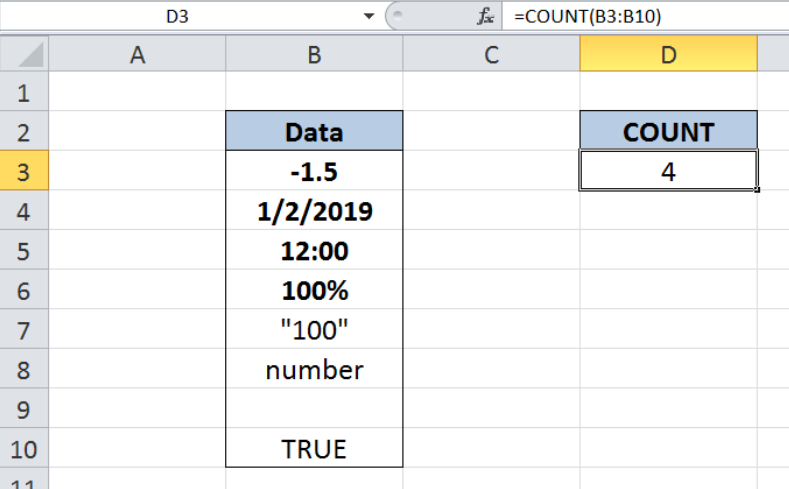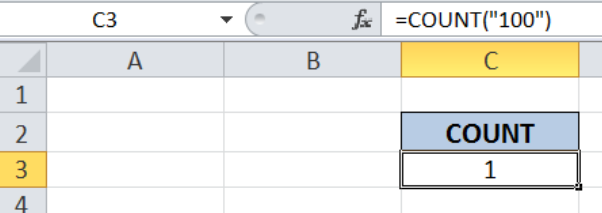Get instant live expert help with Excel or Google Sheets“My Excelchat expert helped me in less than 20 minutes, saving me what would have been 5 hours of work!”

#### Post your problem and you’ll get expert help in seconds.

Your message must be at least 40 characters
Our professional experts are available now. Your privacy is guaranteed.

# How to Use the Count Function in Excel

While working with Excel, we are able to count only the numbers in a data set by using the COUNT function.  This step by step tutorial will assist all levels of Excel users in the usage and syntax of COUNT function.Figure 1. Final result: Using COUNT function in Excel

Final formula:  `=COUNT(B3:B10)`

## Syntax of COUNT Function

COUNT function counts the number of cells containing numbers, including cells that can be represented as numbers such as date and time

`=COUNT(value1, [value2], ...)`

Parameters:

• value –  any value, cell reference or range for which we want to count numbers; only value 1 is required, succeeding values are optional
• Numbers, time and dates are counted, while empty cells, text and logical values are ignored
• Numbers enclosed in quotation marks that are directly typed as arguments are counted

## Setting up Our Data

We have a list in column B consisting of numbers, date, time, empty cell, text and logical values.  We want to count the cells containing numbers by using the COUNT function. The result will be recorded in cell D3.Figure 2.  Sample data in using COUNT function in Excel

## Count cells containing numbers using COUNT

In order to count the cells containing numbers, we follow these steps:

Step 1.  Select cell D3

Step 2.  Enter the formula: `=``COUNT(B3:B10)`

Step 3: Press ENTERFigure 3.  Entering the formula to count numbers using COUNT

The argument for our COUNT function is the range B3:B10, which contains our list.  COUNT function identifies the numbers only, including the values that represent numbers such as date and time.

In our list, the cells counted as numbers are B3, B4, B5 and B6.  As a result, the value in cell D3 is “4”, which is the count for -1.5, 1/2/2019, 12:00, 100%.

The other values are not counted because those are not considered as numbers. Cell B7 is considered as a text value because it is enclosed in quotation marks.  Cells B8, B9 and B10 contain a text, empty cell and logical value, respectively. Hence, cells B7:B10 are ignored by the COUNT function.

## Note

• Numbers enclosed in quotation marks can be counted by the COUNT function when directly typed into the formula.  For example, enter this formula in a cell:
`=COUNT(“100”)`Figure 4.  Number enclosed in quotation marks counted by COUNT function

• We can use COUNTA function when we want to count text, error or logical values
• We can use the COUNTIF or COUNTIFS function when we want to count values based on certain criteria

Most of the time, the problem you will need to solve will be more complex than a simple application of a formula or function. If you want to save hours of research and frustration, try our live Excelchat service! Our Excel Experts are available 24/7 to answer any Excel question you may have. We guarantee a connection within 30 seconds and a customized solution within 20 minutes.

### Did this post not answer your question? Get a solution from connecting with the expert.Another blog reader asked this question today on Excelchat:
Solution examplesI have a column of percentages (11 in a column). I want to have a percentage at the bottom of the column that tells me how many of the entries are = to 100%.
Solved by X. E. in 20 minsI have a spreadsheet that contains a list of names/IDs and I want to generate a formula that inserts into a cell the number of times a name or ID appears on that list. Either insert a column into that spreadsheet or transfer that info into a column on a different spreadsheet. Either way would be fine.
Solved by O. L. in 18 minsneed help.... SUM(COUNTIFS('Parts NC Entry'!\$E\$2:\$E\$1934,M9,'Parts NC Entry'!\$K\$2:\$K\$1934,"X")+COUNTIF('Parts NC Entry'!\$E\$2:\$E\$1934,N9,'Parts NC Entry'!\$K\$2:\$K\$1934,"X"))-COUNTIFS('Parts NC Entry'!\$E\$2:\$E\$1934,M9,'Parts NC Entry'!\$G\$2:\$G\$1934,"TRIAL/SETUP",'Parts NC Entry'!\$E\$2:\$E\$1934,M9,'Parts NC Entry'!\$K\$2:\$K\$1934,"X")-COUNTIFS('Parts NC Entry'!\$E\$2:\$E\$1934,N9,'Parts NC Entry'!\$G\$2:\$G\$1934,"TRIAL/SETUP",'Parts NC Entry'!\$E\$2:\$E\$1934,N9,'Parts NC Entry'!\$K\$2:\$K\$1934,"X")
Solved by V. U. in 15 minsneed help SUM(COUNTIFS('Parts NC Entry'!\$E\$2:\$E\$1934,M9,'Parts NC Entry'!\$K\$2:\$K\$1934,"X")+COUNTIFS('Parts NC Entry'!\$E\$2:\$E\$1934,N9,'Parts NC Entry'!\$K\$2:\$K\$1934,"X"))-COUNTIFS('Parts NC Entry'!\$E\$2:\$E\$1934,M9,'Parts NC Entry'!\$G\$2:\$G\$1934,"TRIAL/SETUP",'Parts NC Entry'!\$E\$2:\$E\$1934,M9,'Parts NC Entry'!\$K\$2:\$K\$1934,"X")-COUNTIFS('Parts NC Entry'!\$E\$2:\$E\$1934,N9,'Parts NC Entry'!\$G\$2:\$G\$1934,"TRIAL/SETUP",'Parts NC Entry'!\$E\$2:\$E\$1934,N9,'Parts NC Entry'!\$K\$2:\$K\$1934,"X")
Solved by O. E. in 10 minsHello, I have a spreadsheet with 3159 rows. One column consists of 9-digit numbers in each of the 3159 rows. I need the formula that can calculate how many of those numbers appear more than once.
Solved by D. H. in 56 mins## Subscribe to Excelchat.coAnother blog reader asked this question today on Excelchat: